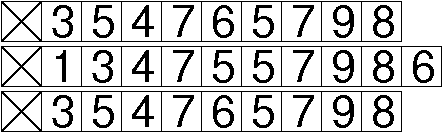CS 61B: Data Structures (Autumn 2010)
Midterm II

### Solutions

Problem 1. (10 points) A Miscellany.

a. Determining whether the graph contains a particular edge (x, y). Other acceptable answers include inserting an edge and deleting an edge. A particularly interesting solution, contributed by one student on his exam, is finding all the edges directed into a particular vertex. This takes O(|V|) time with an adjacency matrix, and O(|V| + |E|) time with an adjacency list.

b.c.e. Three acceptable answers (each worth full points):
If any one of the characters is zero, the hash code is zero.
Anagrams all have the same hash code.
A string's hash code doesn't change if you insert characters equal to one.

Problem 2. (8 points) Algorithms.

a, b. With bottomUpHeap(), create a heap containing the n numbers. This takes Θ(n) time. Then, call removeMin() j times, taking Θ(j log n) worst-case time. The total worst-case running time is in Θ(n + j log n).

An alternative solution uses a max-heap, which reverses the heap-order property, so that the greatest key is at the top of the heap. Starting from an empty max-heap, insert j keys (it doesn't matter which ones) into the heap. Then repeat the following operations for each remaining key: insert the key into the heap, and remove the maximum key from the heap. After you've processed all the keys, the heap contains the j smallest. At worst, this takes Θ(n log j) time.

The optimal solution—which requires knowledge you won't learn until later in the semester—is to use a median-finding algorithm to find the jth-smallest key in Θ(n) expected time. Then, a linear scan through the array can find the other j – 1 smallest items, also in Θ(n) expected time.

c. Output-sensitive.

d. Starting from the root, walk down the tree. When you encounter a key less than k, go right. When you encounter a key greater than or equal to k, go left. Repeat until you reach a null pointer. (A useful intuition is that this is equivalent to performing a find operation on the key k – ε, where ε is infinitesimal.)

As you go down the tree, keep track of the largest key you've encountered so far that's less than k. When you reach a null pointer, return that value. The tree might not contain any key less than k, in which case you can return null or some other value that means “no such key.”

Problem 3. (7 points) Sibling-based tree height.

The main idea is that if this node has a next sibling, then height() should return the maximum height of this node and its siblings to the right. This information allows the parent to calculate its height. The other idea is to use a postorder traversal to calculate the heights.

```public class SibTreeNode {
public Object item;
public SibTreeNode parent;
public SibTreeNode firstChild;
public SibTreeNode nextSibling;

int height() {
int h = 0;
if (firstChild != null) {
h = 1 + firstChild.height();
}
if (nextSibling != null) {
int hSib = nextSibling.height();
if (hSib > h) {
return hSib;
}
}
return h;
}
}
```

Mail inquiries to cs61b@cory.eecs